Test: Amplitude Modulation

# Test: Amplitude Modulation

Test Description

## 10 Questions MCQ Test | Test: Amplitude Modulation

Test: Amplitude Modulation for Electronics and Communication Engineering (ECE) 2023 is part of Electronics and Communication Engineering (ECE) preparation. The Test: Amplitude Modulation questions and answers have been prepared according to the Electronics and Communication Engineering (ECE) exam syllabus.The Test: Amplitude Modulation MCQs are made for Electronics and Communication Engineering (ECE) 2023 Exam. Find important definitions, questions, notes, meanings, examples, exercises, MCQs and online tests for Test: Amplitude Modulation below.
Solutions of Test: Amplitude Modulation questions in English are available as part of our course for Electronics and Communication Engineering (ECE) & Test: Amplitude Modulation solutions in Hindi for Electronics and Communication Engineering (ECE) course. Download more important topics, notes, lectures and mock test series for Electronics and Communication Engineering (ECE) Exam by signing up for free. Attempt Test: Amplitude Modulation | 10 questions in 30 minutes | Mock test for Electronics and Communication Engineering (ECE) preparation | Free important questions MCQ to study for Electronics and Communication Engineering (ECE) Exam | Download free PDF with solutions
 1 Crore+ students have signed up on EduRev. Have you?
Test: Amplitude Modulation - Question 1

### The carrier amplitude after AM varies between 4 volts and 1 volt. The depth of modulation is

Detailed Solution for Test: Amplitude Modulation - Question 1

We know that,
Modulation index;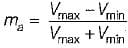Given, Vmax = 4 volt and Vmin  = 1 volt
∴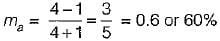Test: Amplitude Modulation - Question 2

### A sinusoidal carrier has an amplitude of 10 V and frequency 30 kHz. It is amplitude modulated by a sinusoidal voltage of amplitude 3 V and frequency 1 kHz. The spectrum of AM wave will be represented as

Detailed Solution for Test: Amplitude Modulation - Question 2

Given,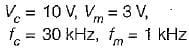∴ Modulation index,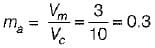The sideband frequencies are as under: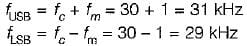Amplitude of each sideband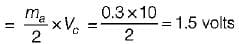Hence, the spectrum of AM wave will be as shown in figure below.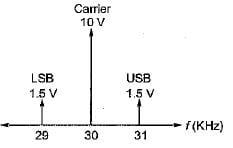Test: Amplitude Modulation - Question 3

### Assertion (A): The principle of envelope detection of an AM signal is based on multiplying the incoming signal with the locally generated synchronous carrier and pass the product through a LPF. Reason (R): An envelope detector produces an output signal that follows the envelope of the input AM signal exactly.

Detailed Solution for Test: Amplitude Modulation - Question 3

The principle of envelope detection of an AM signal is based on rectifying the incoming signal and passing it through a LPF. Hence, assertion is false. Assertion is the principle of synchronous detection.

Test: Amplitude Modulation - Question 4

Consider the following statements associated with the synchronous detection method for detection/demodulation of AM signals:
1. The circuit complexity is very less.
2. It introduces frequency and phase errors only.
3. it does not require any locally generated synchronous carrier.
4. It can be used for DSB-SC, SSB and VSB but not for DSB-FC.
Which of the statements given above is/are correct?

Detailed Solution for Test: Amplitude Modulation - Question 4

• The circuit complexity of synchronous detection is very high. Hence, statement-1 is not correct.
• Statement-2 is correct.
• Synchronous detection require a locally generated synchronous carrier. Hence, statement-3 is not correct.
• Statement-4 is correct because synchronous detection is used for DSB-SC, SSB and VSB while envelope detection is used for DSB-FC only.

Test: Amplitude Modulation - Question 5

Assertion (A): in amplitude modulation, the higher percentage of modulation is preferred.
Reason (R): The modulation index of more than 100% can be achieved without compromising the envelope distortion.
(a) Both A and R are true and R is the correct explanation of A.
(b) Both A and R are true but R is not the correct explanation of A.
(c) A is true but R is false.
(d) A is false but R is true.

Detailed Solution for Test: Amplitude Modulation - Question 5

In amplitude modulation, the higher percentage of modulation is preferred because the higher value of percentage of modulation results into a strong and more intelligible received signal which is less likely to get contaminated or lost in the noise. A modulation index of more than 100% results into over modulation which introduces envelope distortion. Hence, assertion is true but reason is false.

Test: Amplitude Modulation - Question 6

The types of distortions which can occur in the envelope detector output is/are

Detailed Solution for Test: Amplitude Modulation - Question 6

Diagonal Clipping

This type of distortion occurs when the RC time constant of the load circuit is too long. Due to this, the RC circuit cannot follow the fast changes in the modulating envelop.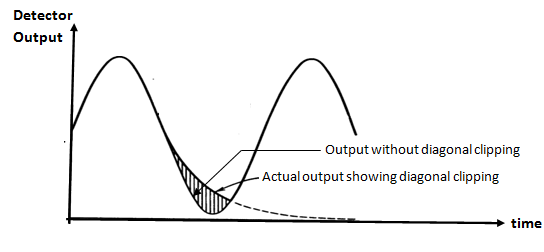Negative Peak Clipping

This distortion occurs due to a fact that the modulation index on the output side of the detector is higher than that on its input side .

Hence, at higher depth of modulation of the transmitted signal, the overmodulation may takes place at the output of the detector .

The negative peak clipping will take place as a result of this overmodulation.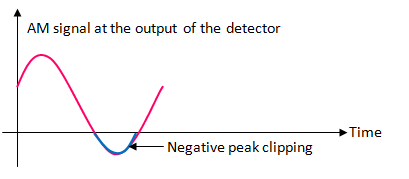Test: Amplitude Modulation - Question 7

A 400 Watts carrier is modulated to a depth of 75 percent. Assuming the modulating signal to a sinusoidal one, the total power in the amplitude-modulated wave is

Detailed Solution for Test: Amplitude Modulation - Question 7

Given,
Pc = 400 Watt, ma = 0.75
The total power in the amplitude modulated wave is: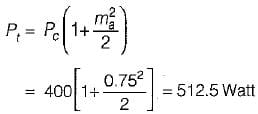Test: Amplitude Modulation - Question 8

The modulation index of the low level emitter modulator is 0.7, voltage gain without modulating signal is AQ = 100, carrier frequency fc = 1 MHz. The amplitude of carrier is 10 mV. If the modulating frequency is 1 kHz, the range of voltage gain is

Detailed Solution for Test: Amplitude Modulation - Question 8

We have: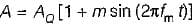or,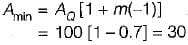and,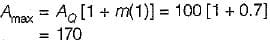Hence, the range of A = 30 to 170

Test: Amplitude Modulation - Question 9

The antenna current of an AM transmitter is 8 A if only the carrier is sent, but it increases to 8.93 A if the carrier is modulated by a single sinusoidal wave. What is the antenna current when the percent of modulation is 80%?

Detailed Solution for Test: Amplitude Modulation - Question 9

Given, Ic = 8 a, ma = 0.8
We know that, antenna current is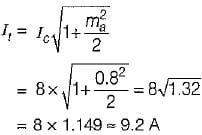Test: Amplitude Modulation - Question 10

The antenna current of an AM transmitter is 10 A when it is modulated to depth of 30% by an audio signal. It increases to 11 A when another signal modulates the carrier signal. The modulation index due to second signal is

Detailed Solution for Test: Amplitude Modulation - Question 10

Given,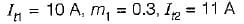We know that, the overall modulation index is: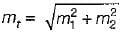or,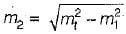Now,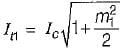or,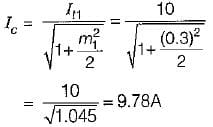After modulating with the second signal, we have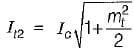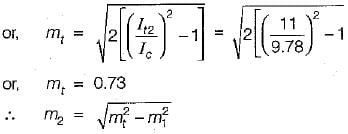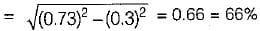Information about Test: Amplitude Modulation Page
In this test you can find the Exam questions for Test: Amplitude Modulation solved & explained in the simplest way possible. Besides giving Questions and answers for Test: Amplitude Modulation, EduRev gives you an ample number of Online tests for practice(Scan QR code)